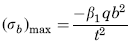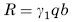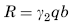Related Resources: calculators

### Three Edges Fixed, One Edge Simply Supported Plate Stress and Reaction Loading Equation and Calculator

Flat Rectangular Plate, Three Edges Fixed, One Edge (a) Simply Supported Loading Over Entire Plate Stress and Deflection Equation and Calculator.

Per. Roarks Formulas for Stress and Strain for flat plates with straight boundaries and constant thickness

Flat Rectangular plate, Three Edges Fixed, One Edge Loading Over Entire Plate

 Rectangular plate; Three Edges FixedAt x = 0, z = 0
StressReaction ForceStress at x = 0, z = 0.6bandAt x = 0, z = b
Reaction ForceAt x = ± a/2, z = 0.6b
StressReaction ForceWhere used:
E = Modulus of Elasticity (lbs/in2)
q = Total load or force to Plate (lbs/in2)
v = Poisson’s ratio (assumed to be 0.3)
t = plate thickness, (in)
a = plate length, (in)
b = plate width, (in)
σb = stress, (lbs/in2)
R = Reaction Force (lbs/in)
β1,2,3,4 = Constant From Table A
γ1,2,3 = Constant From Table A

Table A

 a/b 0.25 0.5 0.75 1 1.5 2 3 β1 0.02 0.081 0.173 0.307 0.539 0.657 0.718 β2 0.004 0.018 0.062 0.134 0.284 0.37 0.422 β3 0.016 0.061 0.118 0.158 0.164 0.135 0.097 β4 0.031 0.121 0.242 0.343 0.417 0.398 0.318 γ1 0.115 0.23 0.343 0.453 0.584 0.622 0.625 γ2 0.123 0.181 0.253 0.319 0.387 0.397 0.386 γ3 0.125 0.256 0.382 0.471 0.547 0.549 0.53

Reference:

Roarks Formulas for Stress and Strain, 7th Edition